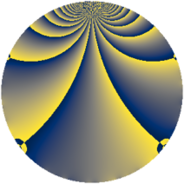# Properties

 Label 1045.2.sLevel $1045$ Weight $2$ Character orbit 1045.s Rep. character $\chi_{1045}(164,\cdot)$ Character field $\Q(\zeta_{6})$ Dimension $232$ Sturm bound $240$

# Related objects

## Defining parameters

 Level: $$N$$ $$=$$ $$1045 = 5 \cdot 11 \cdot 19$$ Weight: $$k$$ $$=$$ $$2$$ Character orbit: $$[\chi]$$ $$=$$ 1045.s (of order $$6$$ and degree $$2$$) Character conductor: $$\operatorname{cond}(\chi)$$ $$=$$ $$1045$$ Character field: $$\Q(\zeta_{6})$$ Sturm bound: $$240$$

## Dimensions

The following table gives the dimensions of various subspaces of $$M_{2}(1045, [\chi])$$.

Total New Old
Modular forms 248 248 0
Cusp forms 232 232 0
Eisenstein series 16 16 0

## Trace form

 $$232 q + 108 q^{4} - 4 q^{5} - 112 q^{9} + O(q^{10})$$ $$232 q + 108 q^{4} - 4 q^{5} - 112 q^{9} - 16 q^{11} - 48 q^{14} + 6 q^{15} - 100 q^{16} - 56 q^{20} + 4 q^{25} + 24 q^{26} - 12 q^{34} + 136 q^{36} - 52 q^{44} - 36 q^{45} + 104 q^{49} - 10 q^{55} - 84 q^{59} + 18 q^{60} - 80 q^{64} - 6 q^{66} + 12 q^{70} + 84 q^{71} - 18 q^{80} - 84 q^{81} - 72 q^{86} + 24 q^{89} - 168 q^{91} + 8 q^{99} + O(q^{100})$$

## Decomposition of $$S_{2}^{\mathrm{new}}(1045, [\chi])$$ into newform subspaces

The newforms in this space have not yet been added to the LMFDB.